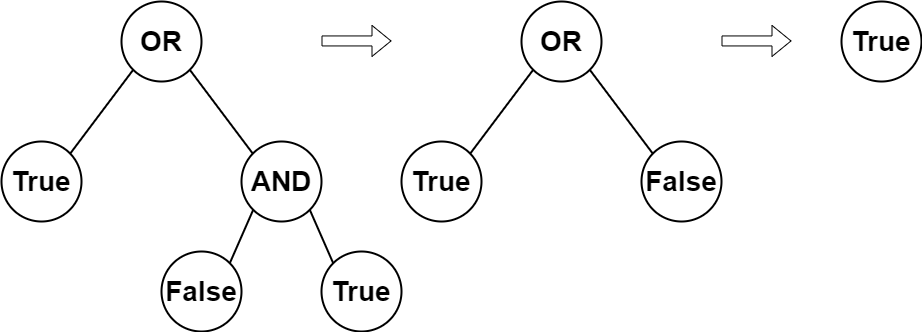# 2331. Evaluate Boolean Binary Tree

Easy
You are given the `root` of a full binary tree with the following properties:
• Leaf nodes have either the value `0` or `1`, where `0` represents `False` and `1` represents `True`.
• Non-leaf nodes have either the value `2` or `3`, where `2` represents the boolean `OR` and `3` represents the boolean `AND`.
The evaluation of a node is as follows:
• If the node is a leaf node, the evaluation is the value of the node, i.e. `True` or `False`.
• Otherwise, evaluate the node's two children and apply the boolean operation of its value with the children's evaluations.
Return the boolean result of evaluating the `root` node.
A full binary tree is a binary tree where each node has either `0` or `2` children.
A leaf node is a node that has zero children.
Example 1:Input: root = [2,1,3,null,null,0,1]
Output: true
Explanation: The above diagram illustrates the evaluation process.
The AND node evaluates to False AND True = False.
The OR node evaluates to True OR False = True.
The root node evaluates to True, so we return true.
Example 2:
Input: root = 
Output: false
Explanation: The root node is a leaf node and it evaluates to false, so we return false.
Constraints:
• The number of nodes in the tree is in the range `[1, 1000]`.
• `0 <= Node.val <= 3`
• Every node has either `0` or `2` children.
• Leaf nodes have a value of `0` or `1`.
• Non-leaf nodes have a value of `2` or `3`.

### 解題

/**
* Definition for a binary tree node.
* type TreeNode struct {
* Val int
* Left *TreeNode
* Right *TreeNode
* }
*/
func evaluateTree(root *TreeNode) bool {
if root.Val == 0 {
return false
} else if root.Val == 1 {
return true
} else if root.Val == 2 {
return evaluateTree(root.Left) || evaluateTree(root.Right)
}
return evaluateTree(root.Left) && evaluateTree(root.Right)
}